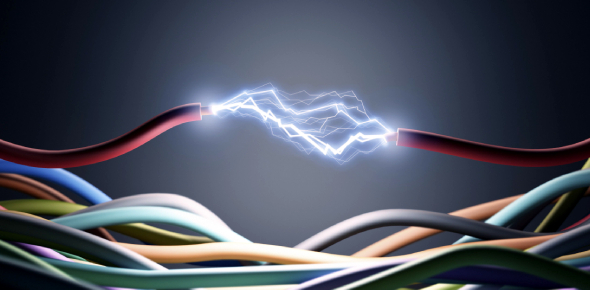# Electrical Energy MCQ Quiz Test!

9 Questions | Total Attempts: 2031SettingsCreate your own QuizHave you ever imagined what is electricity and electrical energy? Do you know how electricity is generated? And from where does it come? Electricity is defined as the flow of electric charge, but that's not enough. There are some questions unanswered. This quiz has been developed for students to test their knowledge about electricity and electrical energy. Let's try it out!

• 1.
Electricity can only flow if a circuit is ___.
• A.

Incomlete

• B.

Open

• C.

Closed

• D.

Simple

• 2.
Look at the picture. When electricity is turned on, which force is produced?
• A.

Magnetic force

• B.

Thermal energy

• C.

Light

• D.

Sound

• 3.
In a radio, electrical energy is changed into ___.
• A.

Light

• B.

Sound

• C.

Thermal

• D.

Magnetic force

• 4.
A D-cell battery is a source of __________________
• A.

Electricity

• B.

force

• C.

magnetism

• D.

Fuel

• 5.
What is a pathway for the flow of electricity?
• A.

Circuit

• B.

Wire

• C.

Tunnel

• D.

None

• 6.
What provides the energy to light a light bulb?
• A.

Energy field

• B.

Magnetic field

• C.

Circuit board

• D.

Electricity source

• 7.
In a simple series circuit, why does the bulb light when you close the switch?
• A.

The switch produces electricity

• B.

Closing the switch completes the circuit

• C.

closing the switch breaks the circuit

• D.

None

• 8.
If there is a 1.5V battery and a bulb on a simple series circuit and the battery is changed to a 3V, what happens to the bulb?
• A.

It gets dimmer

• B.

It gets brighter

• C.

It gets overheated

• D.

Nothing happens

• 9.
A lead-acid battery stores what kind of electricity?
• A.

Virtual

• B.

Direct current (DC)

• C.

Static

• D.

Alternating current (AC)

Related TopicsBack to top
×

Wait!
Here's an interesting quiz for you.